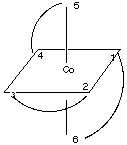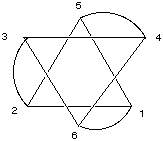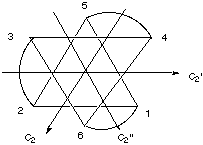Group Theory Solutions: #2

2.* (1997 F 12) The compound tris (ethylenediamine) cobalt (III), Co (H2NCH2CH2NH2) 33-, has the structure pictured below:where the curved lines represent the three bidentate en ligands, each one of which is attached to the Co atom by the N atoms at two points.

Another way to view this stucture is to realize that vertices (1,2,5) and (3,4,6) form triangles (planes) in which 1 is opposite 3, 2 is opposite 4, and 5 is opposite 6:A. Does this molecule possess one or more C2 axes of rotation? If so, where are they and how many are there?B. Does this molecule possess one or more C3 axes of rotation? If so, where are they and how many are there?

One C3 axis. It goes directly into the center of one of the 8 faces of the "octahedron" and comes out the other side. (This isn't an octahedron; it's D3, so it doesn't have the other C3 axes that an octahedron has. You can visualize the D3 point group by stretching or compressing the octahedron along one of its C3 axes. Stretching or compressing in this way breaks the other C3 axes of the octahedron, just as adding the bidentate ligands does.)

C. Does this molecule possess an inversion center?

no

D. Does this molecule possess one or more reflection planes? If so, where are they and how many are there?

no